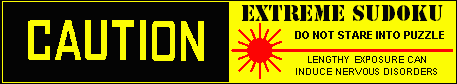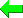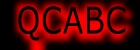Print Version
Solvers
Puzzles
Latest Apps
Str8ts
Other

Discussion...

Post an idea here...
Your Name or Handle

Your comment, idea or question

 Please enter theletters you see:Remember me

Email addresses are never displayed, but they are required to confirm your comments. To state your solve time use the smaller form at the top right of this page." & vbNewLine & _ Line breaks and paragraphs are automatically converted ? no need to use <p> or <br> tags.

... by: SuDokuFan

@numpl_npm if there is no contradiction when you do the proof, it cannot be called an exocet.

... by: numpl_npm

By Andrew's solver with Exocet and DFC( Digit Forcing Chains) only,

+5H5

So +46AB5 and solved.

... by: numpl_npm

GExocet?: 127 HJ6, C4 E5

+7(H6|J6) and -7C4 -7E5 => not contradiction (i.e. solved)

... by: numpl_npm

Sorry
>When +6B7 => +7C7 +1C4 +1F5
--->
>When +6B7 => +7(C7|C9) +1C4 +1F5

... by: numpl_npm

With basics only,

| _ _ _ | _ _ _ | 8 _ _ | A
| _ 8 _ | 5 _ _ | _ _ 1 | B
| 5 _ _ | b 9 8 | c 2 c | C

| a 5 y | _ 7 9 | d 6 d | D
| 6 _ _ | 3 _ 5 | _ _ _ | E
| 7 _ _ | _ _ _ | _ _ 2 | F

| _ _ 5 | _ 8 6 | e 7 e | G
| 8 x _ | _ 5 _ | 2 _ _ | H
| 9 7 _ | 4 3 z | _ _ _ | J

(x in 134) => (x in y)
> When (1 in x) => (1 not in a) (1 in b) (1 in ee) (1 not in dd) (1 in y)
> When (3 in x) => (3 not in a) (3 in cc) (3 in ee) (3 not in dd) (3 in y)
> When (4 in x) => (4 not in a) (4 in cc) (4 in ee) (4 not in dd) (4 in y)

So (28 not in y)

(2 in z) => contradiction(with basics only)
So (1 in z)

...

+6(B5|B7) => +1F5
>When +6B5 => +1F5
>When +6B7 => +7C7 +1C4 +1F5

...

... by: Frans Goosens

@James Havard:
You are right, below the good sudoku.

With trial and error

Combination B1=234 and G9=349

************************************************************
B1=2 G9=3 No solution, Fixed

Combination 2-digits cells

B5=4 No solution, Undo calculation
B5=6 Wrong, Undo calculation
B5=4 No solution, Fixed
B6=3 No solution, Undo calculation
B6=7 Wrong, Undo calculation
B6=3 No solution, Fixed
B3=6 Wrong, Undo calculation
B3=7 No solution, Fixed
C9=4 Wrong, Undo calculation
C9=7 Wrong, Undo calculation

All reset to initial position

************************************************************
B1=2 G9=4 No solution, Fixed

Combination 2-digits cells

B5=4 No solution, Undo calculation
B5=6 Wrong, Undo calculation
B5=4 No solution, Fixed
B6=3 No solution, Undo calculation
B6=7 Wrong, Undo calculation
B6=3 No solution, Fixed
B3=6 Wrong, Undo calculation
B3=7 No solution, Fixed
C9=3 Wrong, Undo calculation
C9=7 Wrong, Undo calculation

All reset to initial position

************************************************************
B1=2 G9=9 No solution, Fixed

Combination 2-digits cells

B5=4 No solution, Undo calculation
B5=6 Wrong, Undo calculation
B5=4 No solution, Fixed
A1=3 Wrong, Undo calculation
A1=4 Wrong, Undo calculation

All reset to initial position

************************************************************
B1=3 G9=3 Wrong, Undo calculation

All reset to initial position

************************************************************
B1=3 G9=4 No solution, Fixed

Combination 2-digits cells

B8=4 Wrong, Undo calculation
B8=9 No solution, Fixed
D9=3 Wrong, Undo calculation
D9=8 No solution, Fixed
E2=1 Wrong, Undo calculation
E2=2 Wrong, Undo calculation

All reset to initial position

************************************************************
B1=3 G9=9 No solution, Fixed

Combination 2-digits cells

B6=2 Wrong, Undo calculation
B6=4 Wrong, Undo calculation

All reset to initial position

************************************************************
B1=4 G9=3 No solution, Fixed

Combination 2-digits cells

B5=2 Wrong, Undo calculation
B5=6 Wrong, Undo calculation

All reset to initial position

************************************************************
B1=4 G9=4 Wrong, Undo calculation

All reset to initial position

************************************************************
B1=4 G9=9 ( I6=1 ) Solved,

#369--------------------------Solution
000 000 800-------------129 743 856
080 500 001-------------487 562 931
500 098 020-------------563 198 427

050 079 060-------------251 879 364
600 305 000-------------694 325 718
700 000 002-------------738 614 592

005 086 070-------------345 286 179
800 000 200-------------816 957 243
970 430 000-------------972 431 685

Total solving time is : 616 sec.
Number of logical steps is : 60833

... by: Neil

This puzzle is very similar to 359.

... by: numpl_npm

Sorry

(6 in tt) (y in tt) => (6 in rr) (y in rr)

... by: numpl_npm

With basics only,

| _ _ _ | _ _ _ | 8 _ _ | A
| _ 8 _ | 5 _ _ | _ _ 1 | B
| 5 _ a | b 9 8 | c 2 c | C

| _ 5 _ | d 7 9 | e 6 f | D
| 6 u _ | 3 _ 5 | _ _ _ | E
| 7 v _ | _ _ _ | _ _ 2 | F

| x _ 5 | _ 8 6 | _ 7 _ | G
| 8 _ _ | _ 5 _ | 2 g _ | H
| 9 7 _ | 4 3 _ | _ _ _ | J

(1 in uv) => (1 in x)
>When (1 in a) => (1 in x)
>When (1 in b) => (1 in e) (1 in x)

(2 in u) => (2 in d) (2 in x)

(3 in v) => (3 in x)
>When (3 in a) => (3 in x)
>When (3 in cc) => (3 in ef) (3 in g) (3 in x)

(4 in uv) => (4 in x)
>When (4 in a) => (4 in x)
>When (4 in cc) => (4 in ef) (4 in g) (4 in x)

So (only one of 1234) in uv, and (9 in uv), and ...

| z p _ | _ _ _ | 8 _ _ | A
| z 8 _ | 5 _ _ | _ _ 1 | B
| 5 p a | b 9 8 | c 2 c | C

| y 5 _ | d 7 9 | e 6 f | D
| 6 u _ | 3 _ 5 | _ _ _ | E
| 7 v _ | _ _ _ | _ _ 2 | F

| x q 5 | _ 8 6 | _ 7 _ | G
| 8 q r | _ 5 _ | 2 g _ | H
| 9 7 r | 4 3 _ | _ _ _ | J

(zz = qq) (6 in pp) (y in pp) (6 in tt) (y in tt).

... by: BobW

@SuDokuFan
You are correct that my solver doesn't implement forcing chains. The only chaining technique that my solver uses is probably closest to Nishios (with a bit of Bowman Bingo thrown in).

It assumes one cell candidate to be true and then propagates singles eliminations in the cells visible to it. Each of these candidates then propagates singles eliminations as well. This is carried out until one of the following happens:
- A contradiction is found (a cell has no remaining candidates, or a house has no position available for one of the digits) which indicates the original hypothesis in invalid and the hypothesis candidate can be eliminated from the initial cell.
- All cells are filled in with digits with no contradictions, so a successful solution has been found.
- All possible eliminations have been made without any contradictions, but not all cells are solved, so this is inconclusive and does not yield any eliminations.

I chose this method for two reasons. Firstly, it's very efficient to solve by computer (all branches of nets are solved in parallel, and the maximum depth of search is known at the outset). Secondly, it's a method that a human player could easily perform with pencil and paper using elimination pencil ticks.

It works very well generally, but from what I've noted from this week's puzzle, it misses some eliminations that would be found with regular forcing chains. And vice versa, I guess. More work to be done.

... by: James Havard

@Frans Goosens Did you allow that the puzzle has the clue J5=3? With J5=0, the puzzle has 12 solutions.

... by: Cenoman

The Junior Exocet must be used further its initial eliminations. Solution with JE2 and AICs only.

1. JE2 (1234) AB1, D3, G2
Eliminations -28D3, -9EF3, -6H2 (2D3: False in mirror node; 8D3 & 6H2: non base digit in target cells; 9EF3: non base digit 8 locked in mirror node)

At that point the following AIC is available:
2. (8)E3=F3-F4=(8-2)D4=(2)E5 =>-2E3
That means: 2 is False in mirror node EF3. Then it can't be True either in the opposite target cell G2. 2 can be True in none of the target cells. Therefore it is a false base digit. =>-2AB1, -2G2

From there, puzzle solved with AICs, e.g. the following sequence:
3. (2)A2=E2-E5=(2)AB5 =>-2A46
4. (6)C2=(6-2)A2=E2-D1=(2-8)D4=(8-6)F4=F5-B5=(6)B7 =>-6C79
5. (8)D9=(8-2)D4=G4-J6=(2-6)J3=J79-H9=(56)AJ9 =>-8J9
6. (9)A3=B3-(9=347)B8.C79-C3=(79)AB3 =>-12A3
7. (2)E5=E2-A2=(2*-1)A5=EF5-(1=4)F6 =>-4E5, -2B5*
8. (7)E7=(7-8)E9=E3-F3=(8-6)F4=F5-B5=(6)B7 =>-7B7
9. (9=347)B8.C79-C3=(7-2)B3=J3-(2=179)H46.J6 =>-9H8
10. (9)H9=H4-(9=2)G4-(2=156)J679 =>-6H9
11. 5-link bivalue oddagon (34)B1, C37, G17 with a single guardian => +1G7; singles to 81
Last step using a Deadly Pattern can be replaced with AICs.

... by: SuDokuFan

@BobW
Reason your solver probably cant proceed is because it doesnt use forcing chains, as far as Ive seen, the remaining solve requires quite a few forcing chains

... by: Frans Goosens

Met -trial and error-

Combinatie B1=234 en G9=349

************************************************************
B1=2 G9=3 Geen oplossing, Gefixeerd

Combinatie 2-cijferige cellen

D9=4 Geen oplossing, Ongedaan gemaakt.
D9=8 Fout, Ongedaan gemaakt.
D9=4 Geen oplossing, Gefixeerd
C9=6 Geen oplossing, Ongedaan gemaakt.
C9=7 Geen oplossing, Ongedaan gemaakt.
D1=1 Fout, Ongedaan gemaakt.
D1=3 Fout, Ongedaan gemaakt.

Alles teruggezet naar begin stand

************************************************************
B1=2 G9=4 Geen oplossing, Gefixeerd

Combinatie 2-cijferige cellen

D9=3 Geen oplossing, Ongedaan gemaakt.
D9=8 Fout, Ongedaan gemaakt.
D9=3 Geen oplossing, Gefixeerd
C9=6 Geen oplossing, Ongedaan gemaakt.
C9=7 Geen oplossing, Ongedaan gemaakt.
D1=1 Fout, Ongedaan gemaakt.
D1=4 Fout, Ongedaan gemaakt.

Alles teruggezet naar begin stand

************************************************************
B1=2 G9=9 Geen oplossing, Gefixeerd

Combinatie 2-cijferige cellen

B6=3 Fout, Ongedaan gemaakt.
B6=4 Fout, Ongedaan gemaakt.

Alles teruggezet naar begin stand

************************************************************
B1=3 G9=3 ( D9=4 ) Opgelost,

#369--------------------------Oplossing
000 000 800-------------129 734 856
080 500 001-------------387 562 491
500 098 020-------------564 198 327

050 079 060-------------253 879 164
600 305 000-------------641 325 789
700 000 002-------------798 641 532

005 086 070-------------415 286 973
800 000 200-------------836 917 245
970 400 000-------------972 453 618

Totale oplostijd is: 266 sec.
Aantal logische stappen is : 27514

... by: BobW

My solver found the same Jexocet as others, but couldn't solve without a second (generic) exocet and then 3 short inference chains:

JExocet: 1234 AB1, D3 G2; Eliminations -28D3 -6H2 -9E3 -9F3

GExocet: 127 HJ6, C4 E5; Eliminations -6A4 -6C4 -4E5

JExocet: 134 AB1, D3 G2; Eliminations -23G2

Inference Chain/Net:
J9=6? > (A9=5 > A8=4 > C7=3 > D7=4 > D3=*1r)&(A9=5 > A8=4 > C7=3 > C3=4) > [Column 3]∌3 ∴ J9≠6

Inference Chain/Net:
E8=4? > (B8=3 > B1=4 > G1=3)&((B8=3 > C7=4D7=*1b) > G7=*3c > G1≠3) > G1=? ∴ E8≠4

Inference Chain/Net:
D7=1? > (D3=3 > F2=9 > H2=*3c)&(E8=9 > H8=*1c)&(D3=3 > D9=4 > H9=3) > [Row H]∌4 ∴ D7≠1

Then singles to the end.

... by: numpl_npm

By Andrew's Solver with Exocet CFG(Cell Forcing Chains) UFC(Unit Forcing Chains) only,

+5H5

So +39EF2 and solved.

... by: James Havard

20 subs in 28 seconds
2 subs together to get a basics solution B6=2 and C3=3

... by: SuDokuFan

JExocet (1234) AB1, D3, G2
Eliminations -28D3, -9EF3, -6H2
Then solvable

Archive

Each week a new 'unsolvable' will be published and the previous will be accessible here from this archive section. If you like very tough puzzles, these are for you.
Share and Enjoy

Page created on 03-May-2011, last modified on 03-May-2011.
All puzzles on this site are trademarked and copyright
and cannot be reproduced without permission.
Copyright Syndicated Puzzles Inc, 2011-2015